## Friday, 19 August 2022

### Use of Proprietary Software in the Aid Sector Perpetuates Racial Injustice

A real thought provoking article arrived in in-box this morning from the brilliant Suchith Anand and just highlights the usual bias by large corporations that if you pay for software it must by definition be better than a free alternative in every case. The initiative just proves there should be no cost barrier to innovation. https://ivangayton.net/2020/07/03/use-of-proprietary-software-in-the-aid-sector-perpetuates-racial-injustice/

## Tuesday, 31 May 2022

### Introduction to 'R' packages 'mapsf' and 'tmap'

‘R’ Mapping Demo - ‘mapsf’ and ‘tmap’
``````library(tmap)
data("World")

tm_shape(World) +
tm_polygons("HPI")``````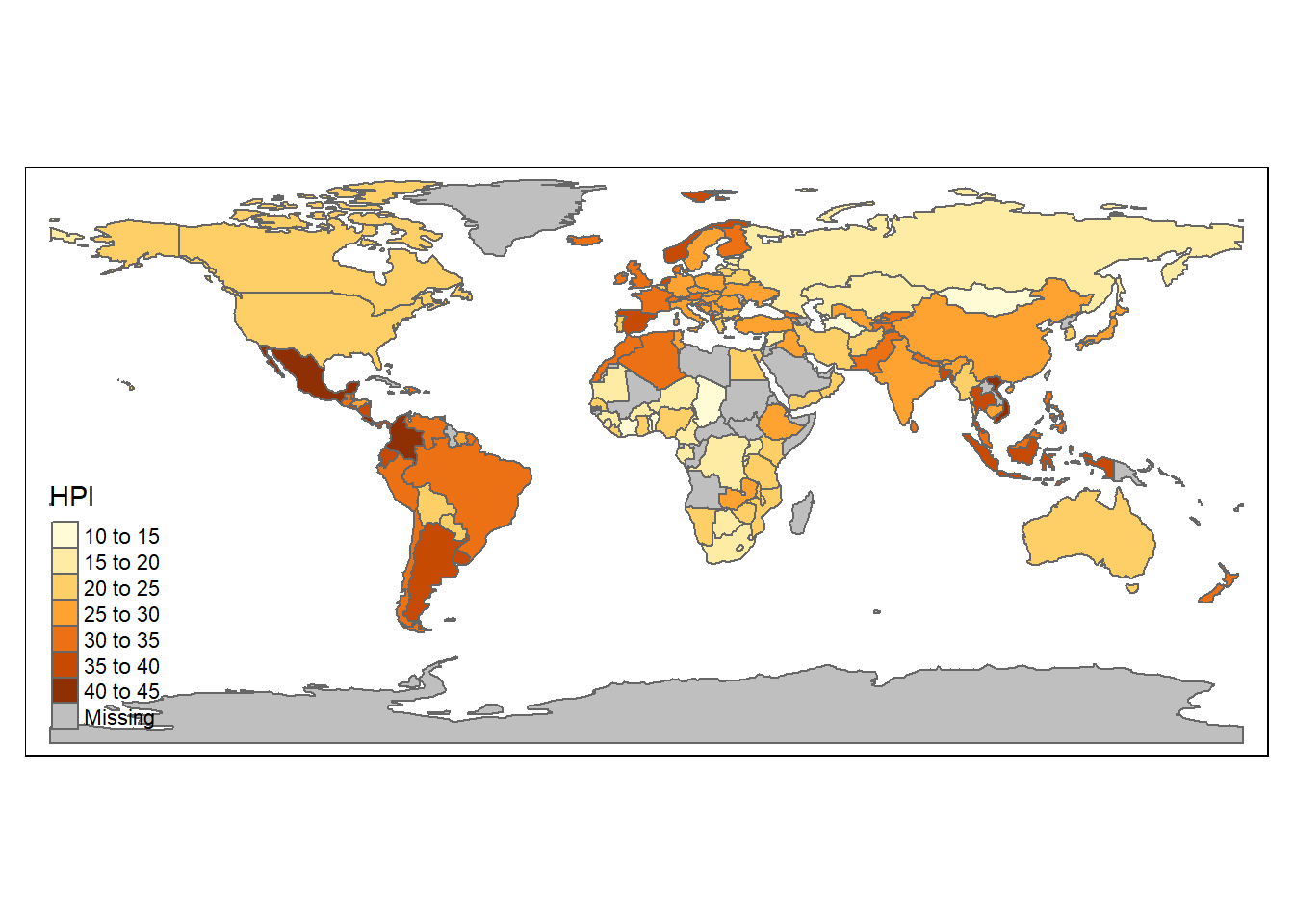``````# install.packages("cartography")
library(sf)``````
``## Warning: package 'sf' was built under R version 4.1.3``
``## Linking to GEOS 3.9.1, GDAL 3.2.1, PROJ 7.2.1; sf_use_s2() is TRUE``
``library(cartography)``
``````## This project is in maintenance mode.
## Core functionalities of `cartography` can be found in `mapsf`.
## https://riatelab.github.io/mapsf/``````
``````# path to the geopackage file embedded in cartography
path_to_gpkg <- system.file("gpkg/mtq.gpkg", package="cartography")
# import to an sf object
mtq <- st_read(dsn = path_to_gpkg, quiet = TRUE)
# plot municipalities (only borders are plotted)
plot(st_geometry(mtq), col = "grey80", border = "grey")
# plot population
propSymbolsLayer(
x = mtq,
var = "POP",
inches = 0.25,
col = "brown4",
legend.pos = "topright",
legend.title.txt = "Total population"
)
# layout
layoutLayer(title = "Population Distribution in Martinique",
sources = "Sources: Insee and IGN, 2018",
author = paste0("cartography ", packageVersion("cartography")),
frame = FALSE, north = FALSE, tabtitle = TRUE)
# north arrow
north(pos = "topleft")``````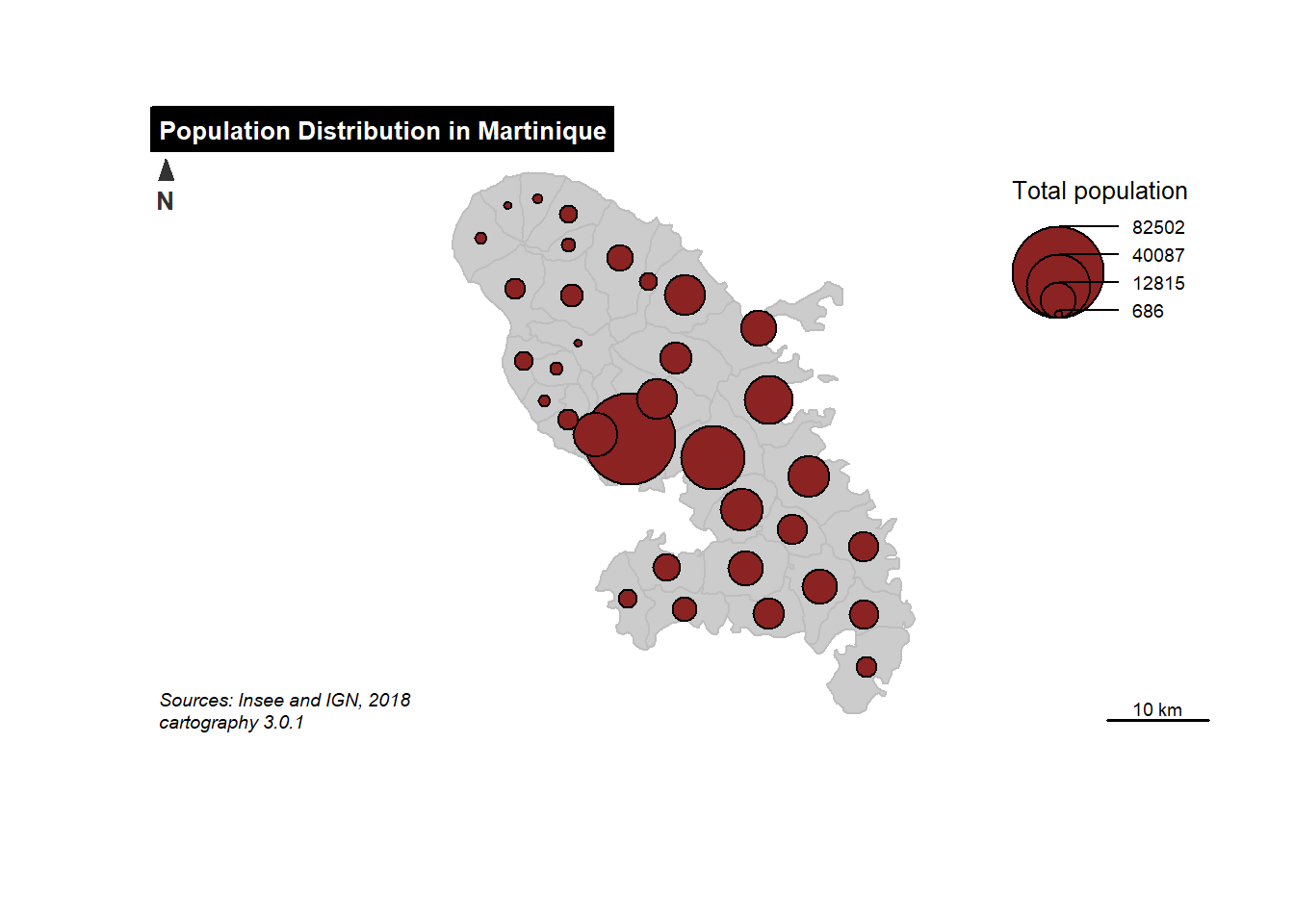``````library(sf)
library(cartography)
# path to the geopackage file embedded in cartography
path_to_gpkg <- system.file("gpkg/mtq.gpkg", package="cartography")
# import to an sf object
mtq <- st_read(dsn = path_to_gpkg, quiet = TRUE)
# population density (inhab./km2) using sf::st_area()
mtq\$POPDENS <- 1e6 * mtq\$POP / st_area(mtq)
# plot municipalities (only the backgroung color is plotted)
plot(st_geometry(mtq), col = NA, border = NA, bg = "#aadaff")
# plot population density
choroLayer(
x = mtq,
var = "POPDENS",
method = "geom",
nclass=5,
col = carto.pal(pal1 = "sand.pal", n1 = 5),
border = "white",
lwd = 0.5,
legend.pos = "topright",
legend.title.txt = "Population Density\n(people per km2)",
)
# layout
layoutLayer(title = "Population Distribution in Martinique",
sources = "Sources: Insee and IGN, 2018",
author = paste0("cartography ", packageVersion("cartography")),
frame = FALSE, north = FALSE, tabtitle = TRUE, theme= "sand.pal")
# north arrow
north(pos = "topleft")``````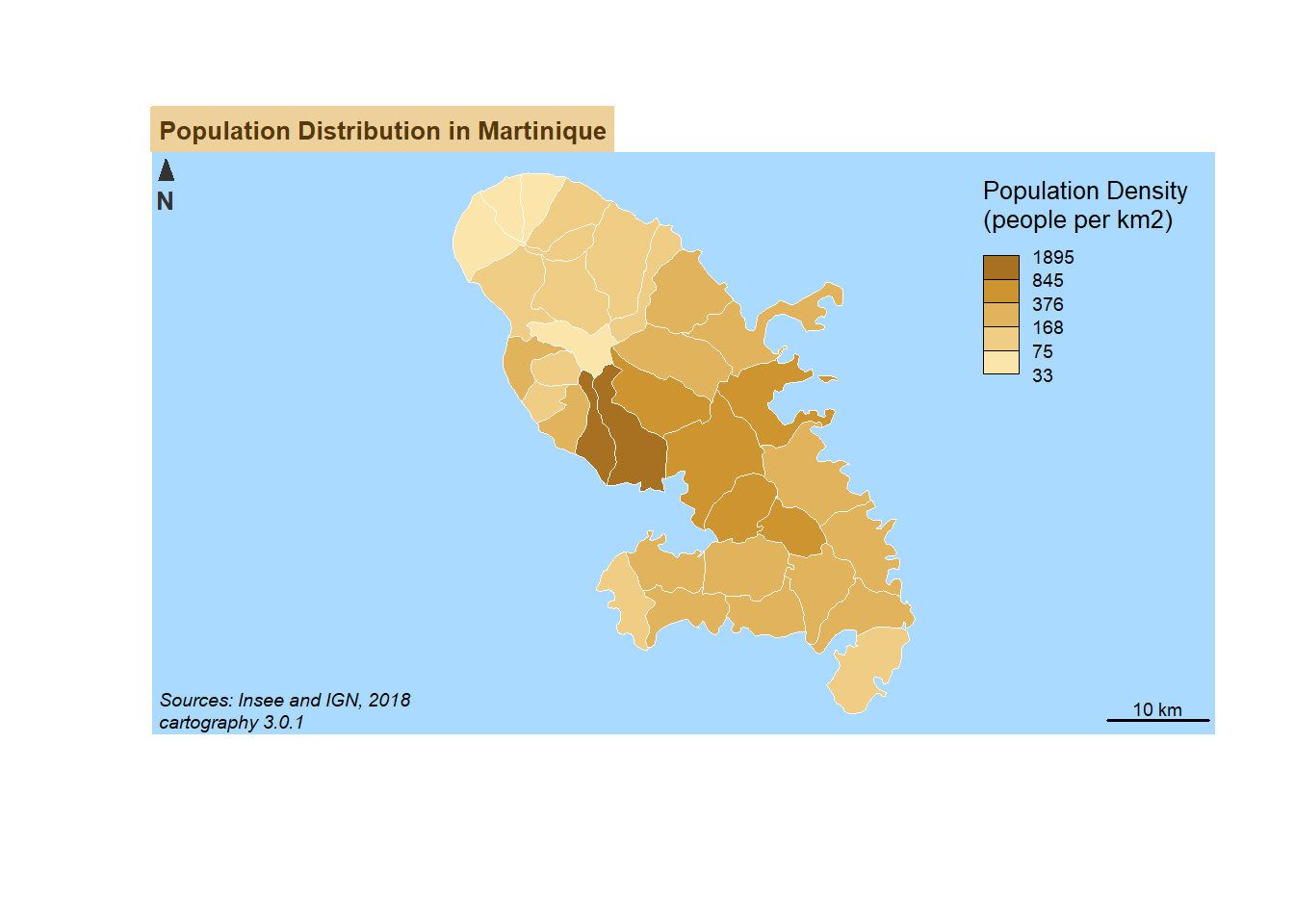``````library(sf)
library(cartography)
# path to the geopackage file embedded in cartography
path_to_gpkg <- system.file("gpkg/mtq.gpkg", package="cartography")
# import to an sf object
mtq <- st_read(dsn = path_to_gpkg, quiet = TRUE)
# transform municipality multipolygons to (multi)linestrings
mtq_pencil <- getPencilLayer(
x = mtq,
size = 400,
lefthanded = F
)
# plot municipalities (only the backgroung color is plotted)
plot(st_geometry(mtq), col = "white", border = NA, bg = "lightblue1")
# plot administrative status
typoLayer(
x = mtq_pencil,
var="STATUS",
col = c("aquamarine4", "yellow3","wheat"),
lwd = .7,
legend.values.order = c("Prefecture",
"Sub-prefecture",
"Simple municipality"),
legend.pos = "topright",
legend.title.txt = "",
)
#  plot municipalities
plot(st_geometry(mtq), lwd = 0.5, border = "grey20", add = TRUE, lty = 3)
# labels for a few  municipalities
labelLayer(x = mtq[mtq\$STATUS != "Simple municipality",], txt = "LIBGEO",
cex = 0.9, halo = TRUE, r = 0.15)
# title, source, author
layoutLayer(title = "Administrative Status",
sources = "Sources: Insee and IGN, 2018",
author = paste0("cartography ", packageVersion("cartography")),
north = FALSE, tabtitle = TRUE, postitle = "right",
col = "white", coltitle = "black")
# north arrow
north(pos = "topleft")``````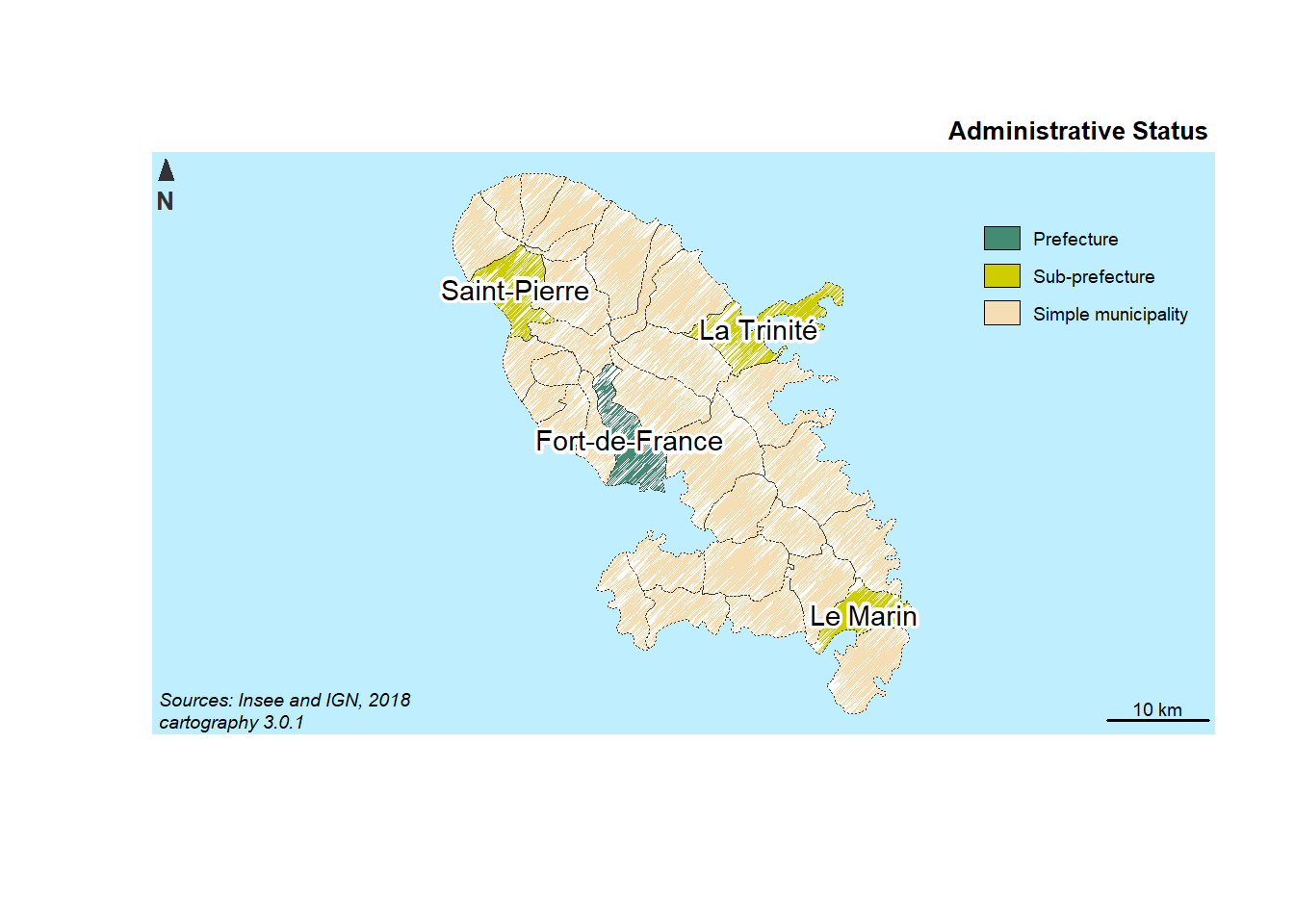``````library(sf)
library(cartography)
# path to the geopackage file embedded in cartography
path_to_gpkg <- system.file("gpkg/mtq.gpkg", package="cartography")
# import to an sf object
mtq <- st_read(dsn = path_to_gpkg, quiet = TRUE)
# Plot the municipalities
plot(st_geometry(mtq), col="darkseagreen3", border="darkseagreen4",
bg = "lightblue1", lwd = 0.5)
# Plot symbols with choropleth coloration
propSymbolsChoroLayer(
x = mtq,
var = "POP",
border = "grey50",
lwd = 1,
legend.var.pos = "topright",
legend.var.title.txt = "Population",
var2 = "MED",
method = "equal",
nclass = 4,
col = carto.pal(pal1 = "sand.pal", n1 = 4),
legend.var2.values.rnd = -2,
legend.var2.pos = "left",
legend.var2.title.txt = "Median\nIncome\n(in euros)"
)
# layout
layoutLayer(title="Population & Wealth in Martinique, 2015",
author = "cartography 2.1.3",
sources = "Sources: Insee and IGN, 2018",
scale = 5, tabtitle = TRUE, frame = FALSE)
# north arrow
north(pos = "topleft")``````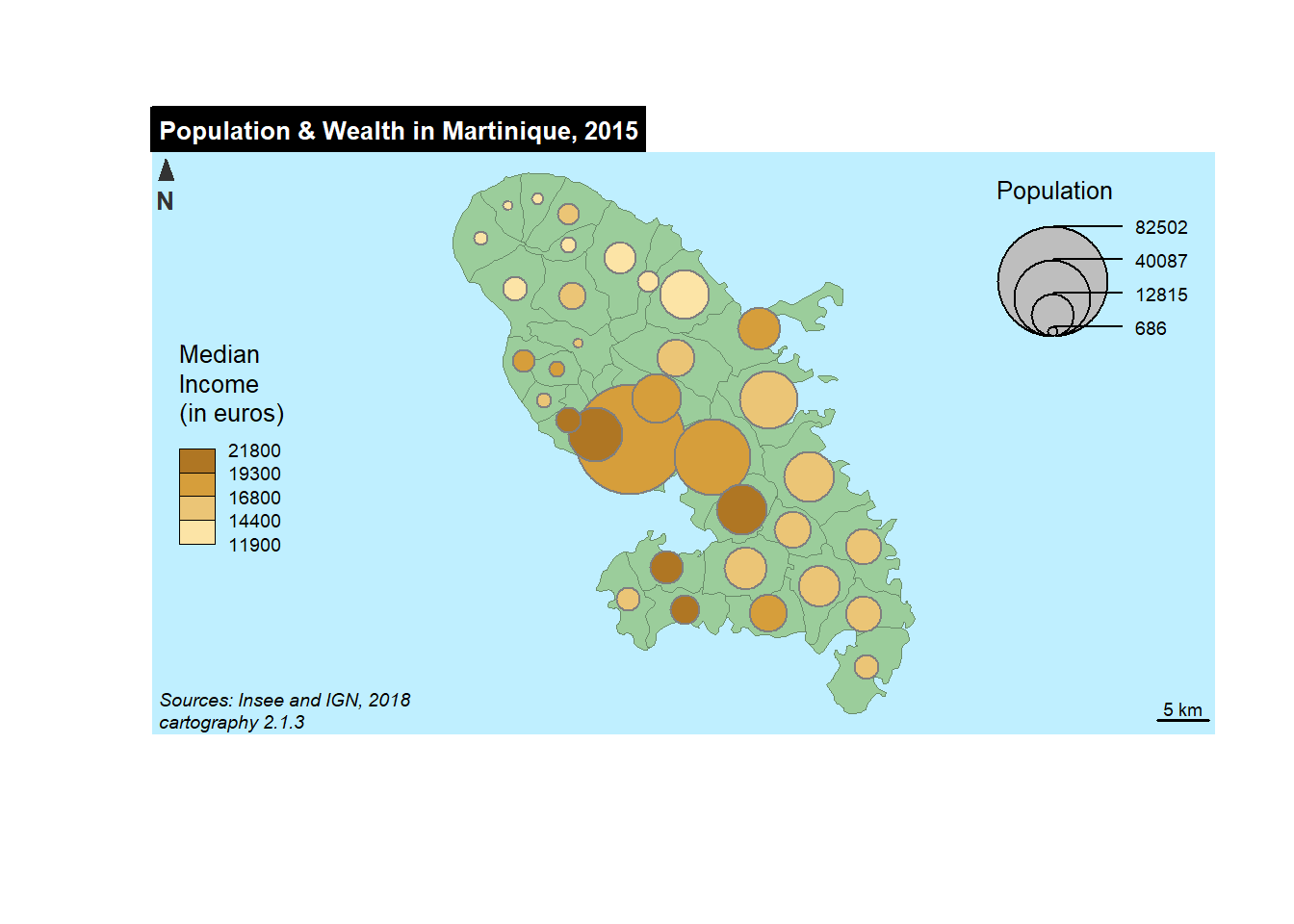``````library(sf)
library(cartography)
# path to the geopackage file embedded in cartography
path_to_gpkg <- system.file("gpkg/mtq.gpkg", package="cartography")
# import to an sf object
mtq <- st_read(dsn = path_to_gpkg, quiet = TRUE)
# Plot the municipalities
plot(st_geometry(mtq), col="#f2efe9", border="#b38e43", bg = "#aad3df",
lwd = 0.5)
# Plot symbols with choropleth coloration
propSymbolsTypoLayer(
x = mtq,
var = "POP",
inches = 0.5,
symbols = "square",
border = "white",
lwd = .5,
legend.var.pos = "topright",
legend.var.title.txt = "Population",
var2 = "STATUS",
legend.var2.values.order = c("Prefecture", "Sub-prefecture",
"Simple municipality"),
col = carto.pal(pal1 = "multi.pal", n1 = 3),
legend.var2.pos = c(692000, 1607000),